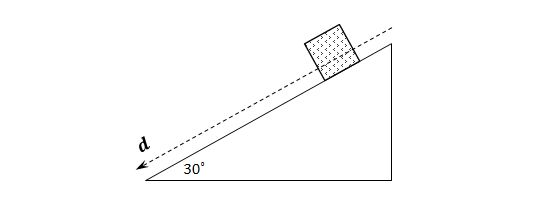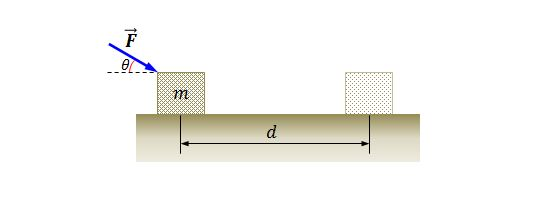Classical Mechanics

# Work-energy theorem (vector form)A constant force of $35\text{ N}$ is applied to an object at an angle of $-45^\circ$ with the horizontal as shown above. The object is pulled $12\text{ m}$ at an angle of $15^\circ$ with the horizontal. How much work is done in the process of moving the object?

A box with a mass of $20\text{ kg}$ is moved along a horizontal floor by a worker. He is pulling on the box with a rope. The angle between the rope and the floor is $30^\circ.$ The tension in the rope is $250\text{ N}$ and the box slides a distance of $10\text{ m}.$ Approximately how much work is done on the box by the rope?

Notes

• $\sqrt{3}$ is about $1.732.$The above diagram depicts a ramp of distance $d=10\text{ m}$ with an incline angle of $30^\circ.$ A box with mass $m=25\text{ kg}$ is sliding down the inclined plane and the coefficient of kinetic friction between the box and the ramp is $\mu_k=0.2.$ What is the total work done on the box by all of the forces acting on it?

The gravitational acceleration is $g=10\text{ m/s}^2.$

Four different objects are being acted on by four respective forces of identical magnitudes and different directions. The directions of each force make angles of $84^{\circ}, 53^{\circ}, 38^{\circ},$ and $2^{\circ},$ respectively, with the horizontal axis. If the horizontal displacements of the four objects are equal after the forces have been applied, which of the following options correctly compares the amount of work, $W_{\theta},$ done by the different forces?A block is pushed for a distance of $d=2.7\text{ m}$ along a frictionless horizontal floor by a constantly applied force of magnitude $F=16.0\text{ N}.$ The mass of the block is $m=2.5\text{ kg}$ and the angle between the applied force and the floor is $\theta=30^\circ,$ as shown in the above figure. Approximately how much work is done on the box by the applied force?

$\sqrt{3}$ is about $1.732.$

×